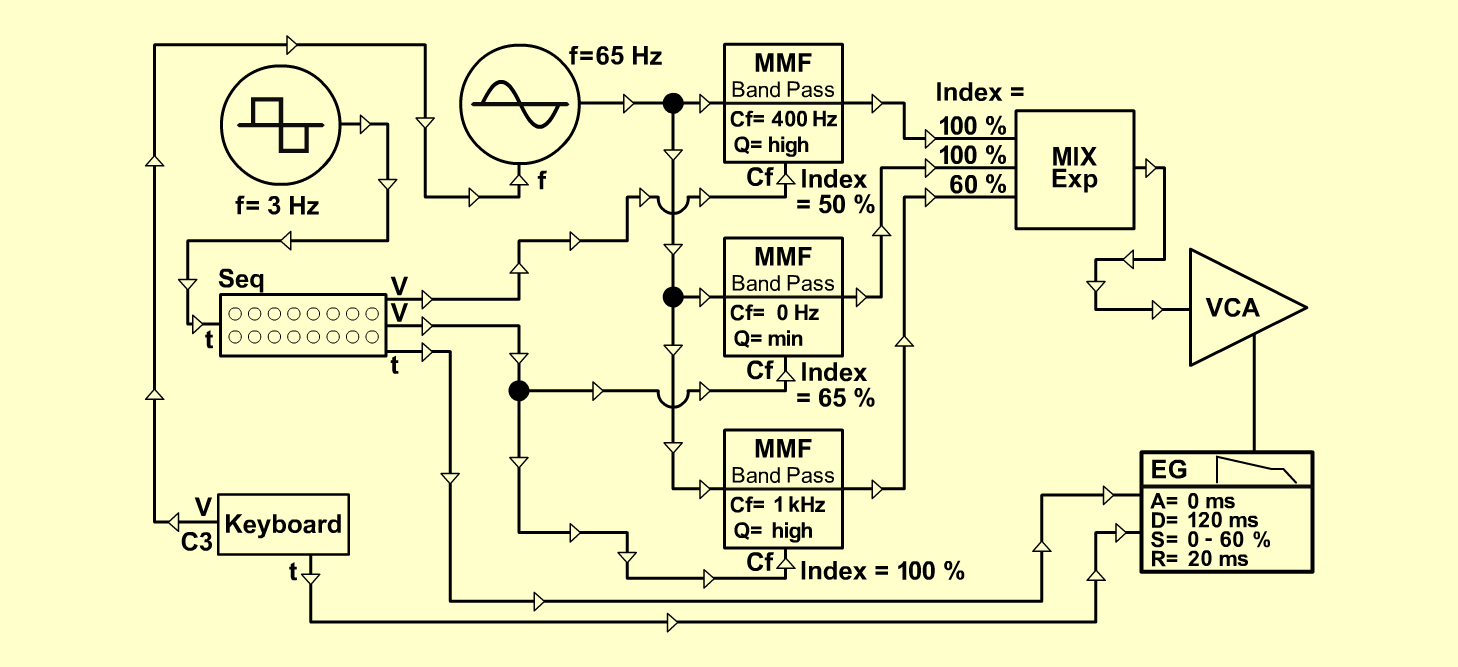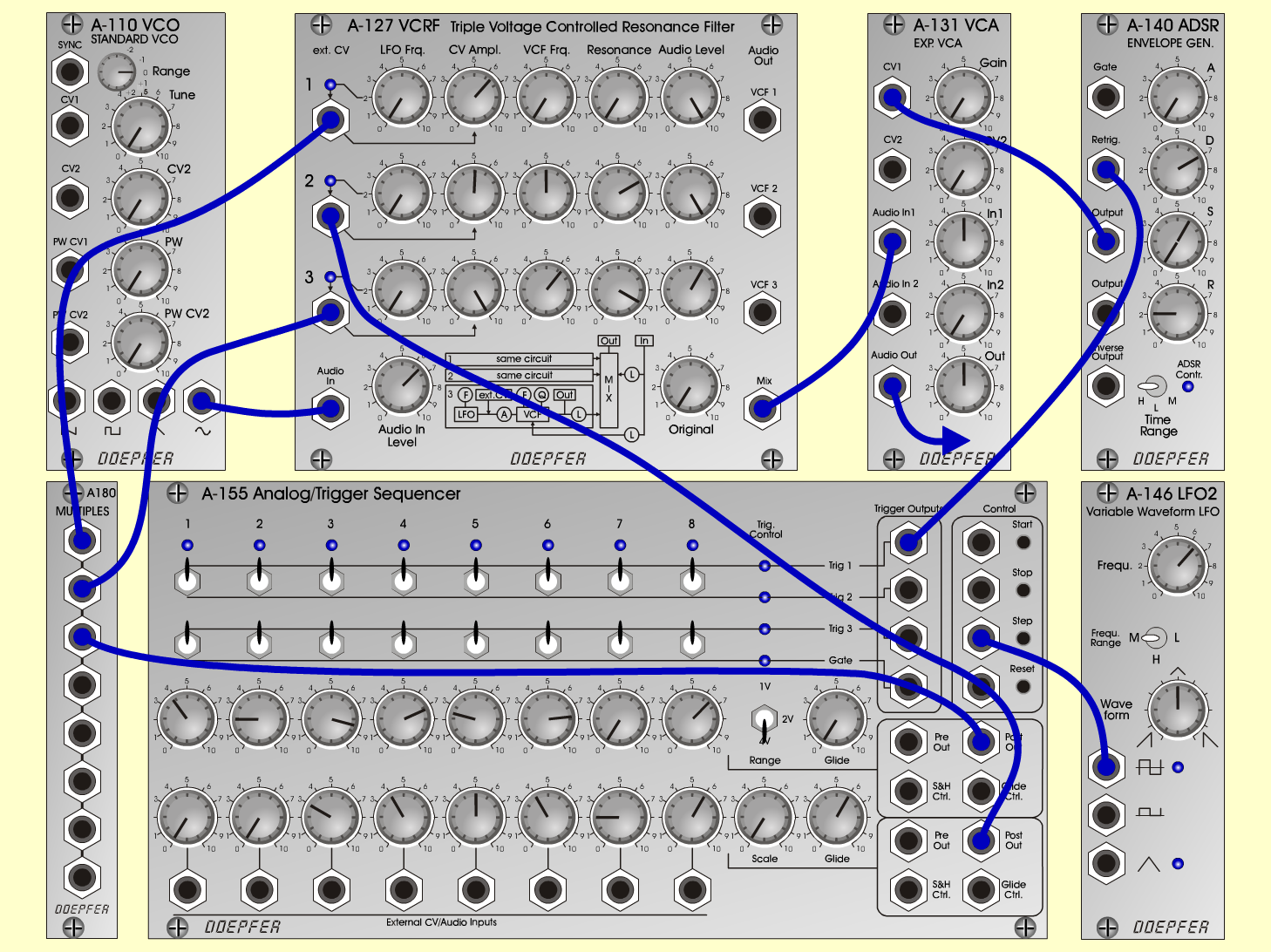Reso-TickerTextureD o e p f e r A - 100 Connections: Settings: A-110 (Sine)  <=>  A-127 (Audio In) A-127 (Mix)  <=>  A-131 (Audio In) A-146 (Pulse)  <=>  A-155 (Step/Clock) A-155 (Trig 1)  <=>  A-140 (Retrig) A-155 (Post Out 1)  <=>  A-180 A-155 (Post Out 2)  <=>  A-127 (ext. CV 2) A-180  <=>  A-127 (ext. CV 1) A-180  <=>  A-127 (ext. CV 3) Gate: KB Pitch: KB (C3) A-110 (Range = 0) A-127 (Audio In = 6.5, Original = 0; Row 1: LFO Freq = 0, CV Ampl = 6.4, VCF Frq = 0, Res = 0, Audio Level = 10; Row 2: LFO Freq = 0, CV Ampl = 5.1, VCF Frq = 5, Res = 7, Audio Level = 10; Row 3: LFO Freq = 0, CV Ampl = 10, VCF Frq = 6.3, Res = 9, Audio Level = 6) A-131 (Gain = 0, Audio In 1 +  Audio Out = variable) A-140 (A = 0, D = 7, S = var. 0 - 6, R = 2, Range = L) A-146 (Freq = 6.4, Wave Form = 5, Range = M) A-155 (Trigger Row Up: all switches set to Trig 1; Row Up: 1 = 3.8, 2 = 2, 3 = 8.5, 4 = 7.2, 5 = 2.5, 6 = 7.8, 7 = 0, 8 = 6.5, Range = 4V, Glide = 0/ Row Down: 1 = 0, 2 = 0, 3 = 3, 4 = 4, 5 = 5, 6 = 4, 7 = 2, 8 = 6, Scale = 10, Glide = 6 Notes: I used a tube preamp with a little tube compression afterwards the A-100. Variations can be done by playing with the values of the sequencers second row. Try this patch with a little bit of reverb on it! Manuel G. Richter, April 2002Sound samples Reso-Ticker (with tube compression) Reso-Ticker (with tube comp. and reverb)